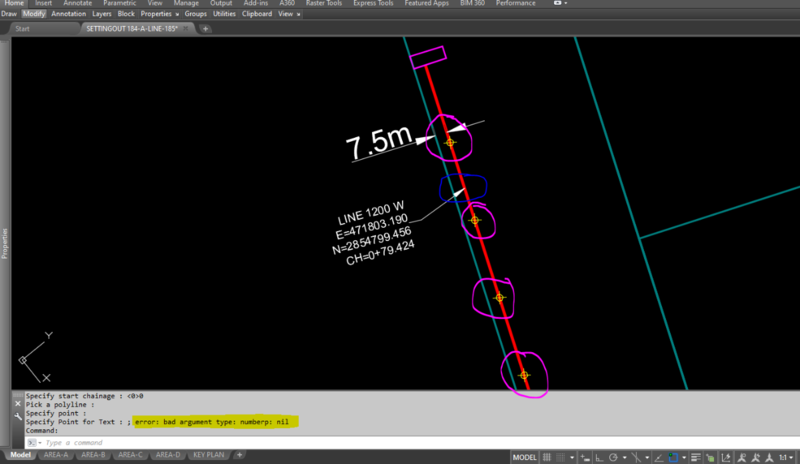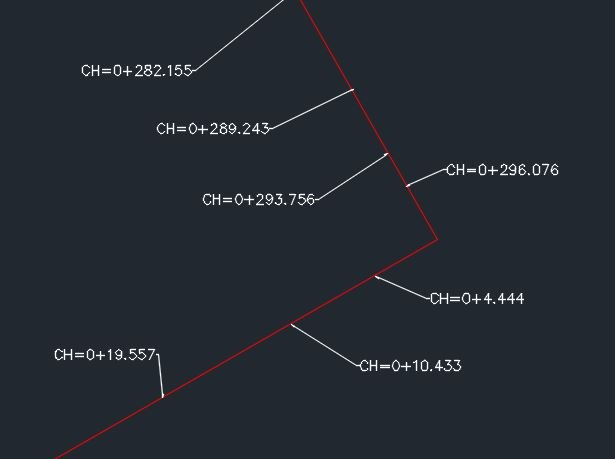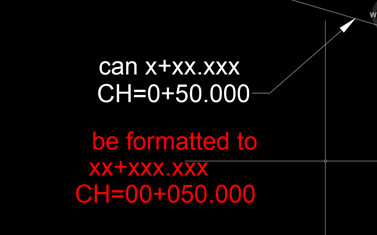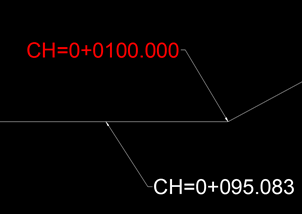# To get length of polyline from start to upto where i click## Recommended PostsHai, i need a help in editing a lisp code. I have a working lisp routine which gives me perpendicular distance to main pline when clicked on a place perpendicular to the pline i selected. Only thing i want to change is i dont need perpendicular distance from main pline but i need same main pline distance(chainage) where i click.

Pls reply if its possible... thanks:)

Attaching the lisp file and also adding same lisp code below,

```(defun c:CE (/ s p c a d tp sch)
(setvar "cmdecho" 0)
(setq sch (getstring "\nSpecify start chainage : <0>"))			;"0" is set as the default value
(if (= sch "")(setq sch "0"))
(if
(and (setq s (car (entsel "\nPick a polyline :")))
(or (= (cdr (assoc 0 (entget s))) "LWPOLYLINE")
)
)
(while
(and
(setq
p (getpoint "\nSpecify point perpendicular to polyline :")
)
(setq c (vlax-curve-getclosestpointto s p))
(setq a (angle p c))
(not (grdraw p c 1 -1))
;; rubber line in red colour.
(setq d (angle	'(0. 0. 0.)
(vlax-curve-getfirstderiv
s
(vlax-curve-getparamatpoint s c)
)
)
)
(or
(or (equal (rem (+ d (* pi 0.5)) (+ pi pi)) a 1e-4)
(equal (rem (+ d (* pi 1.5)) (+ pi pi)) a 1e-4)
)
"Picked point is not a perpendicular to picked polyline. <!>"
)
)
(setq tp (getpoint "\nSpecify Point for Text : "))
)
(strcat "CH=" sch "+" (rtos (vlax-curve-getdistatpoint s c) 2 3))
(strcat "E=" (rtos (car p) 2 3))
(strcat "N=" (rtos (cadr p) 2 3))
""
)
(vlax-put-property (vlax-ename->vla-object (entlast)) 'height 0.60);;; Text Height 0.60
)
)
(setvar "cmdecho" 1)
(princ)
)

##### Share on other sitesMaybe  this can help you, it's a great program from Lee Mac.

##### Share on other sitesIt is very easy there is a vl function note though it will be always from start of pline. A quicky effort

```(defun c:getdist ( / oldsnap pt ent)
(setq oldsnap (getvar 'osmode))
(setvar 'osmode 512)
(setq ent (entsel "Pick obj"))
(while (setq pt (getpoint "\nPick point Enter to exit"))
(princ (vlax-curve-getdistatpoint (vlax-ename->vla-object (car  ent)) pt))
)
(setvar 'osmode oldsnap)
(princ)
)```

•1
##### Share on other sitesOn 6/3/2021 at 6:18 PM, BIGAL said:

It is very easy there is a vl function note though it will be always from start of pline. A quicky effort

```

(defun c:getdist ( / oldsnap pt ent)
(setq oldsnap (getvar 'osmode))
(setvar 'osmode 512)
(setq ent (entsel "Pick obj"))
(while (setq pt (getpoint "\nPick point Enter to exit"))
(princ (vlax-curve-getdistatpoint (vlax-ename->vla-object (car  ent)) pt))
)
(setvar 'osmode oldsnap)
(princ)
)```

No need to convert the entity to an object, the curve functions are actually faster with enames.

`(vlax-curve-getdistatpoint (car  ent) pt)`
```_\$
Benchmarking ....................Elapsed milliseconds / relative speed for 131072 iteration(s):

(vlax-curve-getDistAtPoint E P)..............1656 / 4.43 <fastest>
(vlax-curve-getDistAtPoint (vlax-ena...).....7344 / 1.00 <slowest>

_\$ ```

Edited by ronjonp
•1
##### Share on other sitesits not working as i wanted, but its giving output, Thank You.

i have a lisp code here, its working in polyline but not points above it. when i use measure command to the polyline, the points are created . when i pick the points to get the chainage ,its showing error. is it possible to get polyline length even if i click the points above it? Please check if possible. other than that the lisp is working wonderfully. hak_vz helped in it.

```(defun c:CE (/ s p c tp sch f)
(setvar "cmdecho" 0)
(setq sch (getstring "\nSpecify start chainage : <0>"))			;"0" is set as the default value
(if (= sch "")(setq sch "0"))
(if
(and (setq s (car (entsel "\nPick a polyline :")))
(or (= (cdr (assoc 0 (entget s))) "LWPOLYLINE")
)
)
(while
(and
(setq
p (getpoint "\nSpecify point :")
)
(setq c (vlax-curve-getclosestpointto s p))
(setq tp (getpoint "\nSpecify Point for Text : "))
)
(setq di (vlax-curve-getdistatpoint s p))
(setq f (fix (/ di 1000.0)))
(setq di (- di (*  f 1000.0)))
(strcat "CH=" (itoa (+ (atoi sch) f)) "+" (rtos di 2 3))
""
)
(vlax-put-property (vlax-ename->vla-object (entlast)) 'height 0.60);;; Text Height 0.60
)
)
(setvar "cmdecho" 1)
(princ)
)```##### Share on other sites1 hour ago, minejash said:

its not working as i wanted, but its giving output, Thank You.

i have a lisp code here, its working in polyline but not points above it. when i use measure command to the polyline, the points are created . when i pick the points to get the chainage ,its showing error. is it possible to get polyline length even if i click the points above it? Please check if possible. other than that the lisp is working wonderfully. hak_vz helped in it.

```
(defun c:CE (/ s p c tp sch f)
(setvar "cmdecho" 0)
(setq sch (getstring "\nSpecify start chainage : <0>"))			;"0" is set as the default value
(if (= sch "")(setq sch "0"))
(if
(and (setq s (car (entsel "\nPick a polyline :")))
(or (= (cdr (assoc 0 (entget s))) "LWPOLYLINE")
)
)
(while
(and
(setq
p (getpoint "\nSpecify point :")
)
(setq c (vlax-curve-getclosestpointto s p))
(setq tp (getpoint "\nSpecify Point for Text : "))
)
(setq di (vlax-curve-getdistatpoint s p))
(setq f (fix (/ di 1000.0)))
(setq di (- di (*  f 1000.0)))
(strcat "CH=" (itoa (+ (atoi sch) f)) "+" (rtos di 2 3))
""
)
(vlax-put-property (vlax-ename->vla-object (entlast)) 'height 0.60);;; Text Height 0.60
)
)
(setvar "cmdecho" 1)
(princ)
)```

REPLACE THIS

`(setq di (vlax-curve-getdistatpoint s p))`

WITH THIS

`(setq di (vlax-curve-getdistatpoint s c))`•1
##### Share on other sitesThank you. it works well now... Thanks

##### Share on other sitesIts a great code, Thanks all for this. I have a small request to make

can the text be further formatted to 00+000.000 instead of 0+00.000

##### Share on other sitesIts a great code, Thanks all for this. I have a small request to make

can the text be further formatted to 00+000.000 instead of 0+00.000

REPLACE this

(if (= sch "")(setq sch "0"))

With this

(if (= sch "")(setq sch "00"))

##### Share on other sites41 minutes ago, hosneyalaa said:

REPLACE this

(if (= sch "")(setq sch "0"))

With this

(if (= sch "")(setq sch "00"))

hosneyalaa, thanks for your help. But its not showing any difference in results.

##### Share on other siteshosneyalaa, thanks for your help. But its not showing any difference in results.

And

REPLACE

(strcat "CH=" (itoa (+ (atoi sch) f)) "+" (rtos di 2 3))

With

(strcat "CH=" (itoa (+ (atoi sch) f)) "+0" (rtos di 2 3))

##### Share on other sites22 minutes ago, hosneyalaa said:

And

REPLACE

(strcat "CH=" (itoa (+ (atoi sch) f)) "+" (rtos di 2 3))

With

(strcat "CH=" (itoa (+ (atoi sch) f)) "+0" (rtos di 2 3))Here the values less than 100 are good, but 100 and above are not true...

Thanks for all your time and efforts..

## Join the conversation

You can post now and register later. If you have an account, sign in now to post with your account.
Note: Your post will require moderator approval before it will be visible.×   Pasted as rich text.   Restore formatting

Only 75 emoji are allowed.